Next: order preserving mapping Up: Definitions Previous: orbit

# order

Let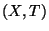be a topological space, and let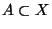. Let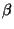be a cardinal number. We say that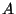is of order less than equal toin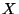, written ord(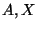)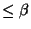, provided that for each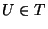such that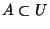, there exists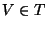such that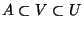and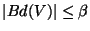. We say thatis of orderin, written ord()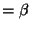, provided that ord()and ord()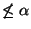for any cardinal number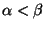. A concept of an order of a point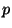in a continuum(in the sense of Menger-Urysohn), written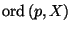, is defined as follows. Let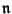stand for a cardinal number. We write: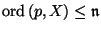provided that for every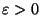there is an open neighborhood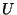ofsuch that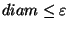and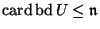;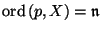provided thatand for each cardinal number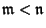the condition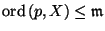does not hold;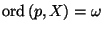provided that the pointhas arbitrarily small open neighborhoodswith finite boundaries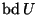and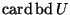is not bounded by any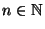.

Thus, for any continuumwe have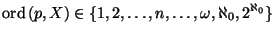(convention: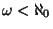); see [Kuratowski 1968, §51, I, p. 274]. Let a dendroidand a point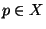be given. Thenis said to be a point of order at least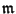in the classical sense provided thatis the center of an-od contained in. We say thatis a point of orderin the classical sense provided thatis the minimum cardinality for which the above condition is satisfied (see [Charatonik 1962, p. 229]).Next: order preserving mapping Up: Definitions Previous: orbit
Janusz J. Charatonik, Pawel Krupski and Pavel Pyrih
2001-11-30